## 3.3.4 Calling of module MA2D3E

The direct call to module MA2D3E  (see also the preprocessor MA23XX) is done by writing a main program as follows:

• dimension array M (in the blank common)
• declare the real array ZINT(0:NBSEC) where NBSEC is the total number of sections (this array could be used totally or partially)
• declare the integer array DESREF(1:5,1:NBDES) where NBDES is the number of physical transfer attributes to effect
• declare the integer array NUCOU(1:3,1:NBFONC) where NBFONC is the number of geometrical transformations to effect
• declare the real array MAT(1:4,1:4,1:NBFONC) of transformation matrices (this array could be used totally or partially)
• declare the logicals BFONC, COLLER and RAPIDE
• declare the externals XYZ23 and XYZ33
• call INITI
• call the module

CALL MA2D3E (M,M,NFNOPE,NINOPE,NFNOPS,NINOPS,
. NBCOU,EPS,NBFONC,NUCOU,MAT,COLLER,RAPIDE,
. DESREF,NBDES,ZINT,BFONC,XYZ23,XYZ33)

where:

• M is the super array
• NF(NI)NOPE is the support number of the 2D I.D.S. NOPO and its level
• NF(NI)NOPS is the support number of the 3D I.D.S. NOPO and its level
• NBCOU is the number of mesh layers (i.e the number of sections - 1 )
• EPS is the threshold of degeneracy (in percentage) to verify the volumes (see RAPIDE)
• NBFONC is the number of geometric transformations to describe
• NUCOU(1:3,1:NBFONC) is the description of the transformations
• NUCOU(1,I) is the starting section number
• NUCOU(2,I) is the arrival section number
• NUCOU(3,I) is the transformation code or TTYPE with (see above):
-1: i-1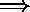i via MAT
-2: 2Dp to q via XYZ23
-3: i-1i via XYZ33
-4: pp+1 to q via ZINT(p+1,..,q)
-5: p and q (XYZ23)p+1 to q via a constant step determined automatically
-6: p and q' (XYZ23)p+1 to q via steps given in
 ZINT(p+1,..,q)
• MAT(1:4,1:4,1:NBFONC): if NUCOU(3,I) = -1, we supply matrix MAT(4,4,I) defining the transformation
• COLLER: .TRUE. if section 0 and section NBCOU+1 are identical and to be glued together, .FALSE. if not
• RAPIDE: .FALSE. if we ask to verify the volumes at EPS percent,, .TRUE. if we ask to verify the length of the vertical edges with the same type of tolerance
• NBDES is the number of physical transfer attributes to effect
• DESREF(1:5,1:NBDES) is the description of these transfers (see above)
• ZINT(.) is the array of sectional sides useful for ZINT(0) if BFONC = .FALSE. (for the basis) and/or if TTYPE = -4 or -6 (for an arbitrary section).
• BFONC: .TRUE. if the basis is defined by function XYZ23, .FALSE. if it is identical to the 2D mesh and has for side ZINT(0)
• XYZ23  and XYZ33 : two subroutines used according to the case (see BFONC or TTYPE of NUCOU), to be written in the following fashion:

```
SUBROUTINE XYZ23(ISEC,NUMPOIN,NREF,x,y,z,X,Y,Z)
```
where
• ISEC is the index of the section to construct
• NUMPOIN is the number of the 2D generic point m
• NREF is its reference
• x, y and z its coordinates (z has no effect in the case of purely 2D data)
• X, Y and Z are the coordinates calculated from the preceding data
and
```
SUBROUTINE XYZ33(ISEC,NUMPOIN,NREF,x,y,z,X,Y,Z)
```
where
• ISEC is the index of the section to construct
• NUMPOIN is the number of the 2D generic point m
• NREF is its reference
• x, y and z are the coordinates of the 3D point of section ISEC-1 associated to the generic point above
• X, Y and Z are the coordinates calculated from the preceding data (i.e. those of the point of section ISEC)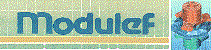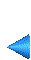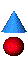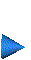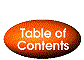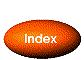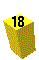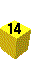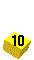Next: 3.3.5 The preprocessor MA23XX Up: 3.3 Extrusion method (cylindrical topology) Prev: 3.3.3 Data definition Index Contents